# What Is Entropy?

Entropy (S) is a thermodynamic quantity originally defined as a criterion for predicting the evolution of thermodynamic systems.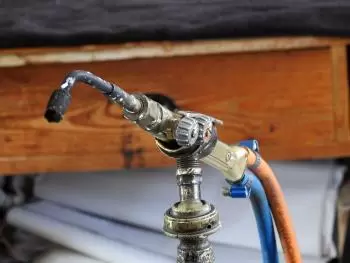Entropy is an extensive state function. The value of this physical magnitude, in an isolated system, grows in the course of a process that occurs naturally. Entropy describes how irreversible a thermodynamic system is.

The word entropy comes from the Greek and means evolution or transformation.

## Entropy in the World of Physics

In physics, entropy is the thermodynamic quantity that allows us to calculate the part of heat energy that cannot be used to produce work if the process is reversible. Physical entropy, in its classical form, is defined by the equation.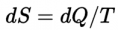or more simply, if the temperature is kept constant in process 1 → 2 ( isothermal process):

Thus, if a hot body at temperature T1 loses a quantity of heat Q1, Its entropy decreases in Q1 / T1, If ​​it gives this heat to a cold body at temperature T2 (less than T1) the entropy of the cold body increases more than the entropy of the hot body has decreased because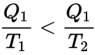A reversible machine can therefore transform part of this heat energy into work, but not all of it.

The performance that the reversible machine gives (which is the maximum that any machine can give) is: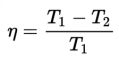In order for all the heat energy to be transformed into work, it would be necessary that either the hot focus be at infinite temperature or that the cold focus be at zero kelvin; otherwise, the thermodynamic performance of the reversible machine is less than 1.

The expression of entropy is a logical consequence of the second principle of thermodynamics and of the way in which temperature is measured.

The second principle of thermodynamics says that, if work is not consumed, the heat from hot bodies to cold bodies, either directly by conduction or through any machine.

The temperature must be measured on a thermodynamic scale; otherwise, the entropy expression is not as elegant and depends on the thermometric substance that is used to build the thermometer. When defining the thermodynamic temperature scale, there is a degree of freedom that can be chosen arbitrarily. If it is imposed that between the boiling temperature and the freezing temperature of the water there are 100 degrees, the Kelvin scale is obtained and it turns out that the freezing temperature of the water must be 273 K.

## Why Is It Not Possible to Know Absolute Entropy?

In the real world it is not possible to determine the absolute entropy of a system why it would be necessary to be able to arrive at absolute 0.

To arrive at absolute 0, the system must first cool to absolute zero so that the molecules no longer move, and in addition, the molecules must be in the most stable state. In that specific case, absolute entropy equals zero (third law of thermodynamics).

Third law of thermodynamics : "The entropy of a perfect crystal approaches zero when T approaches zero (but there are no perfect crystals)."

Changing this steady state from 0 kelvin to the starting point gives the absolute entropy. From here, increasing the temperature will increase the entropy.

Fortunately, from a practical point of view, it is generally sufficient to calculate the entropy variation. Calculating the differences is easier to do experimentally.

The temperature variation is calculated using the heat capacity: the integral of the ratio of heat capacity and temperature over the area of ​​temperature change.

## What Relationship Exists Between Entropy and Energy?

Assuming that the entire universe is an isolated system, that is, a system for which it is impossible to exchange matter and energy with the outside, the first law of thermodynamics and the second law of thermodynamics can be summarized as follows: " the total energy of the universe is constant and the total entropy increases continuously until it reaches equilibrium ”

This means that not only can it not create or destroy energy, nor can it completely transform from one form to another without a part dissipating in the form of hot.

## How and Who Discovered the Concept of Entropy?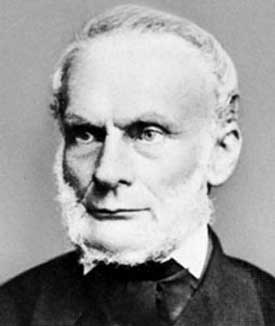The concept of entropy is developed in response to the observation of a certain fact: there is a certain amount of energy released in combustion reactions that is lost due to dissipation or friction. In this way, the energy that is lost is not transformed into useful work.

### Investigations in the First Thermal Engines

Early thermal engines such as the Thomas Savery (1698), the Newcomen engine (1712), and the three-wheeled steam Cugnot (1769) were inefficient. Of the input energy, only 2% was converted into useful energy.

A large amount of useful energy was dissipated or wasted in what seemed like an immeasurable state of randomness.

Over the next two centuries, physicists investigated this puzzle of lost energy.

The result of these studies led scientists to the concept of entropy.

## First Occurrences of the Entropy Concept

Physicist Rudolf Clausius was the first to introduce it in 1865.

Since then various definitions of entropy have appeared. The most relevant definition of entropy is the one developed by Ludwig Boltzmann. Boltzmann relates the concept of entropy to the degree of disorder of a system. This new perspective of entropy allowed the concept to be extended to different fields, such as information theory, artificial intelligence, life or time.

## References

Author:

Published: March 24, 2017
Last review: April 16, 2020